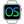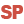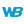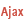WINDEVWEBDEVWINDEV MobileOthersTimeSys (Function)
In french: HeureSys
Returns or modifies the system time (current time defined for the current computer).
Remark: This function is equivalent to Now.
Example
Res = TimeSys()        // Res = "15332105"
Res = TimeToString(TimeSys())   // Res = "15:33:21:05"
CurrentTime = Left(TimeSys(), 4)  // CurrentTime = "1533"// Display the current time in the CurrentTime control

// -- Initialization code of a window/page
// Call the "Clock" procedure every second
TimerSys("Clock", 100, 1)
// -- Clock procedure
PROCEDURE Clock()
CurrentTime = TimeToString(TimeSys())// Changes the system time and displays the new time
TimeSys("17280000")
Info(TimeSys())TodaysDate is Date = DateSys()
EDT_EditDate = DateToDayInAlpha(TodaysDate) + " " + TodaysDate.Day + ...
" " + DateToMonthInAlpha(TodaysDate) + " at " + ...
TimeToString(TimeSys(), "HH:MM:SS")
// The EDT_EditDate control contains for example: Monday 20 August at 14:44:17
Syntax

Getting the system time

<Result> = TimeSys()
<Result>: Character string or Time variable
System time in HHMMSSCC format (HH corresponds to hours, MM to minutes, SS to seconds and CC to hundredths of a second).System time in HHMMSSCCC format (HH corresponds to hours, MM to minutes, SS to seconds and CCC to hundredths of a second).Modifying the system time

TimeSys(<New time>)
<New time>: Character string, Date or DateTime variable
New system time. This time can correspond to:
• a character string in HHMMSSCC format (HH corresponds to hours, MM to minutes, SS to seconds and CC to hundredths of a second).
• a character string in YYYYMMDDHHmmSSCC format (YYYY corresponds to the year, MM to the month, DD to the day, HH to hours, mm to minutes, SS to seconds and CC to hundredths of a second). In this case, only the time is taken into account.
• a Time variable.
• a DateTime variable. In this case, only the time is taken into account.
Remarks
• Caution: To modify the system time, you must have the administrator rights on the current computer.
• To get the time in a format other than HHMMSSCC, the string must be formatted using DateToString, Right, Left, etc.
• The HHMMSSCC format was chosen because it allows you to compare two times with hundredths-of-a-second accuracy.
•The system time corresponds to the current time defined on the server.
•In this version, TimeSys can only be used to return the system time (time on the current computer or on the computer on which the Internet browser is running).
•In simulation mode, the time used is the time on the PC where the simulation is run. At runtime, the time of the mobile device is used.
Related Examples:Unit examples (WINDEV): Calculations on durations [ + ] Calculations on dates and times with the WLanguage functions: - Calculate the duration between two moments (dates and times)- Calculate a sum of durations- Calculate an average of durations
Business / UI classification: Neutral code
Component: wd280std.dll
Minimum version required
• Version 9